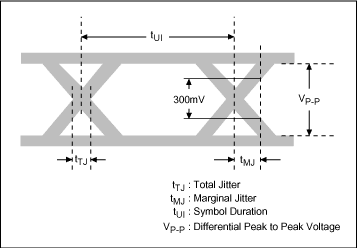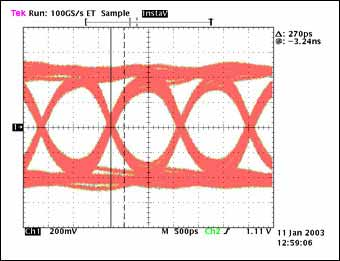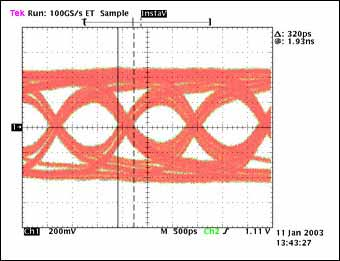# LVDS Serializer-Deserializer Performance over Twisted Pair Cable

A serializer-deserializer pair reduces interconnect wiring in high-bandwidth, short-distance data communications applications such as telecom and networking equipment backplanes, 3G cell phone basestation intra-rack connections, and digital video interfaces. Current-mode LVDS signaling offers simplified termination, low transmission power, and low EMI.

The main LVDS standard, TIA/EIA-644-A, specifies physical layer parameters such as signal levels, but does not specify interconnect performance such as data rate versus cable length. The standard offers users the basic compatibility of LVDS signaling but needs to be supplemented in high data rate applications with information on attainable performance with a given cable type and length.

This article presents laboratory test results and analysis of the bit error ratio (BER) performance of the MAX9205/MAX9207 LVDS serializer and the MAX9206/MAX9208 deserializer at different data rates and cable lengths. This article also correlates BER with the jitter measured on link eye diagrams.

The Maxim MAX9205/MAX9207 LVDS serializer and MAX9206/MAX9208 LVDS deserializer are designed to transmit high-speed data over a serial point-to-point link with 100Ω differential characteristic impedance. The serial "payload" data rate (with overhead synchronization bits excluded) is 160Mbps to 400Mbps for the MAX9205–MAX9206 pair and 400Mbps to 600Mbps for the MAX9207–MAX9208 pair. Both have the same pinout and but each pair is optimized for operation over a different frequency range. The BER and eye diagram jitter using unshielded twisted pair CAT-5E cable of various lengths is investigated.

To measure the reliability of a link, the BER test is the most straightforward and accurate measure. Digital communication links require very low error ratios on the order of one error in one hundred billion received bits (BER of 10-12) or less.

However, to make a BER test, a high performance signal generator and specialized test equipment are needed, and the BER test is time consuming. Depending on the transmission rate, it can take hours or even days to transfer the number of bits required to test for a BER of 10-12 or less. Due to the time consuming nature of a BER test, faster measures are commonly employed to predict link reliability. One is to establish jitter levels that produce a low BER. Jitter for the MAX9205–MAX9206 and MAX9207–MAX9208 links (termed "marginal jitter" in Figure 3) is measured and correlated to BER. The BER test is used to validate the datasheet jitter maximum values.

### Test Setup

The LVDS signal is transmitted by the MAX9205 or MAX9207 LVDS serializer. The serializer latches 10 bits of parallel data on the rising edge of the parallel data clock (TCLK), adds two overhead synchronization bits and transmits the serialized data through a single LVDS output. The parallel data clock range is 16MHz to 40MHz for MAX9205 and 40MHz to 60MHz for MAX9207. With the two synchronization bits included, the serial bit rate is 12 × TCLK. The "payload" serial data rate (serial bit rate minus the two synchronization bits) is 10 × TCLK. The functional blocks of MAX9205/MAX9207 and MAX9206/MAX9208 are shown in Figure 1.In the cable test setup (Figure 2) the serializer and deserializer on evaluation board number two are used to convert the serial I/O of the Agilent 86130A BER tester to parallel. The parallel data is applied to and read from cable test evaluation board number one. The serial data sequence output by the 86130A is 1200 bits long and is generated by taking 1000 bits from a PRBS of 210-1 and inserting "01" synchronization bits every 10 PRBS bits. Insertion of the synchronization bits mimics the bits that would be added by a serializer. The deserializer on board two removes the synchronization bits and presents the PRBS data in parallel to the cable test serializer on board one. The serial data sequence is repeated continuously. The Agilent 81250 generates the required reference clocks (TCLK for the serializer and REFCLK for the deserializer). The cable is Ethernet Category 5E, AWG24, unshielded twisted pair manufactured by General Cable Inc. (part number 2133629H).The BER was tested using cable lengths of 5ft, 15ft, 30ft, 60ft and 100ft. Eye diagram jitter was measured at the deserializer input using a Tektronix TDS784C oscilloscope and Tektronix P6247 1.0GHz differential probe. The delays of the TCLK serializer reference clocks generated by the 81250 were adjusted to meet the serializer input setup and hold time requirements as listed in the datasheet.

### Measurements and Results

Tests were conducted separately for MAX9205–MAX9206 and MAX9207–MAX9208 serializer-deserializer pairs. The serial bit rate generated by the 86130A was 192Mbps–480Mbps for the MAX9205–MAX9206 and 480Mbps–720Mbps for the MAX9207–MAX9208.

To quantify eye diagram signal integrity, two parameters are defined; total jitter tTJ and marginal jitter tMJ. tTJ is the width of the timing jitter measured at zero differential voltage (zero differential voltage is the main horizontal graticle in the oscilloscope plots). tMJ is the time measured from the center of the jitter at zero differential voltage to 300mV peak-to-peak differential voltage (see Figure 3). The deserializer differential input can be roughly assumed to switch at zero volts differential but it is more conservative to assume additional differential voltage to provide the needed overdrive. tTJ assumes switching at zero volts differential but tMJ requires the differential signal to reach 300mVP-P before it is considered to have switched the deserializer input. tMJ is therefore a more conservative measure of signal integrity. tUI (also Figure 3) is defined as the duration of a serial bit (unit interval). The unit interval is the period of the reference frequency divided by 12.

The differential peak-to-peak voltage is denoted as VP-P. VP-P is two times the magnitude of the difference of the single-ended voltages at the measurement point, or VP-P = 2 × |(VOUT+) - (VOUT-)|. For example, at the measurement point, if VOUT+ = 1.35V and VOUT- = 1.10V relative to ground in the high state and VOUT+ = 1.10V and VOUT- = 1.35V relative to ground in the low state, then VP-P = 500mV. Since the measurements are made with a differential probe (which subtracts VOUT- from VOUT+) the eye diagrams show VP-P.Table 1 shows the maximum values of tMJ as specified in the MAX9206/MAX9208 deserializer datasheet. The deserializer is guaranteed to recover data if tMJ is less than or equal to the listed maximum.

 Bit Rate (Mbps) Data Rate (Mbps) Maximum Marginal Jitter tMJ (ps) 192 160 1300 480 400 720 720 600 320

Testing was conducted under two conditions. Under the first condition, tTJ, and tMJ were measured and bit errors counted while running the serial test pattern for one hour for each cable length at the highest data rate allowed for the serializer—deserializer pair under test. Under the second condition, the serial test data was transmitted for more than 10 hours (transmitting more than 1.73 × 1013 bits) under conditions of high jitter such that maximum datasheet values for tMJ were exceeded. tTJ, and tMJ were measured and bit errors counted.

Tables 2 and 3 show test results for cable lengths from 5ft to 60ft for the MAX9205–MAX9206 and MAX9207–MAX9208 serializer-deserializer pairs. The bit rate is the serial signaling rate and the data rate is the "payload" serial data rate (data rate = (10/12) × bit rate).

 Cable Length (Feet) Bit Rate (Mbps) Data Rate (Mbps) Total Jitter tTJ (ps)* Marginal Jitter tMJ (ps)* Differential Voltage VP-P (mV*) Error Count (1.728 x 1012 bits) 5 480 400 200 220 880 No Error 15 480 400 200 260 780 No Error 30 480 400 220 320 636 No Error 60 480 400 360 560 420 No Error *Measurement resolution for tTJ, tMJ is 10ps. Measurement resolution for VP-P is 2mV.
 Cable Length (Feet) Bit Rate (Mbps) Data Rate (Mbps) Total Jitter tTJ (ps)* Marginal Jitter tMJ (ps)* Differential Voltage VP-P (mV*) Error Count (2.592 x 1012 bits) 5 720 600 180 200 852 No Error 15 720 600 180 230 660 No Error 30 720 600 220 270 556 No Error 60 720 600 320 N/A (VP-P < 300mV) 292 No Error *Measurement resolution for tTJ, tMJ is 10ps. Measurement resolution for VP-P is 2mV.

As test examples, Figure 4 shows eye diagrams taken at the MAX9208 deserializer input after 30ft and 60ft of cable.

\To demonstrate the capability of the deserializer to recover data under degraded signal conditions (that is, with less jitter margin than specified in the datasheet) tests were conducted for both serializer-deserializer pairs using 100 feet of cable. The serial test data was transmitted continuously for more than 10 hours. Table 4 shows the measurements of jitter, peak-peak voltage, and error counts. Figure 5 provides the eye diagram plots.

 Cable Length (Feet) Bit Rate (Mbps) Data Rate (Mbps) Total Jitter tTJ (ps)* Marginal Jitter tMJ (ps)* Differential Voltage VP-P (mV*) Error Count (2.592 x 1012 bits) MAX9205–MAX9206 100 480 400 660 N/A 192 Transmitted bits: 1.73 x 1013 Error bits: 0 Test time: > 10hrs MAX9207–MAX9208 100 520 433 1020 N/A 110 Transmitted bits: 1.87 x 1013 Error bits: 0 Test time: > 10hrs *Measurement resolution for tTJ, tMJ is 20ps. Measurement resolution for VP-P is 2mV.No bit errors are recorded in any of the tests. After 100 feet of cable at a bit rate of 520Mbps, the amplitude of the signal is 110mV. This is approximately one third of the 300mVP-P amplitude specified for tMJ. Also, with tTJ = 1020ps, over half the 1923ps unit interval (tUI = 1/520Mbps) is jitter. The error-free test result under these conditions provides a measure of the margin in the datasheet specifications (Table 1).

Also, based on the test results, the BER can be predicted. Assume that the probability of error for any bit in the serial data sequence is the same and the error events are independent among the bits. If the BER is q, then the serial data sequence can be modeled as Bernoulli trials with parameter q. Assuming n is the number of bits transmitted, then probability of an n-bit sequence with no error can be expressed as

Pno error = (1 - q)n

With 100 feet of cable more than 1.73 × 1013 were transmitted with no error. If we assume that the BER value q is less than 3.01 × 10-13, then Pno error given by Eq. 01 is 0.0056. This can be interpreted as if the BER has a value of 3.0 × 10-13 or bigger, the probability of no error occurring for an observed bit sequence of 1.73 × 1013 is 0.0056. In terms of statistics, if a sequence of 1.73 × 1013 bits are transmitted without error the hypothesis of BER < 3.0 × 10-13 is 99.44% correct. Since this confidence is obtained with 100 feet of cable and poor signal quality, shorter cable lengths and better signal quality will produce better confidence in the link reliability.

### Conclusion

Using the BER test, this article has examined the reliability of MAX9205–MAX9206 and MAX9207–MAX9208 serializer-deserializer pairs over low cost CAT-5E cables of various lengths. The results have shown that even with severe signal degradation the link BER is less than 3.0 × 10-13 with more than 99% confidence. The results also demonstrate that the datasheet jitter maximum limits are sufficient and conservative for high link reliability. Since the measurements were conducted under typical conditions, it is recommended that the criterion in Table 1 be used for real applications. This provides margin to compensate for variations in fabrication, supply voltage, and temperature.